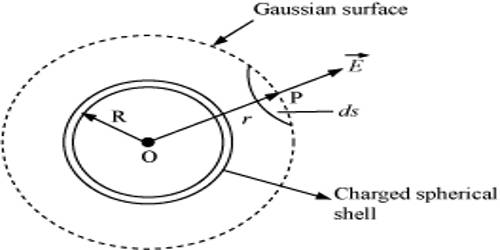Physics

# Gauss’s Law to determine Electric Field due to Charged SphereGauss’s Law to determine Electric field due to charged sphere

The electric field of a sphere of uniform charge density and total charge Q can be obtained by applying Gauss’ law. Let us consider a solid sphere of radius R. If + q amount of charge is given to the sphere, this charge will be distributed uniformly all over the surface of the sphere. No charge will enter into the sphere. Now in order to determine the electric field at a point inside the sphere, a Gauss’s spherical surface of radius r is considered. Due to symmetry, the magnitude of the electric field ‘E’ all over the Gaussian surface will be equal and the direction will be along the radius outwardly. But the Gaussian surface will not certain any charge.So, according to Gauss’s law,

§S E.d . a = §S Eda = E §da = E (4πr2) = 0

then, E = 0

So, inside the sphere i.e., r < R. electric field will be zero.

Again, at points r > R, i.e., for the determination of electric field at any point outside sphere let us consider a spherical surface of radius r [Figure]. Then total charge contained within the confined surface is q.

Again, the electric field E will be of uniform magnitude throughout the Gaussian surface and the direction will be outward along the radius. So, according to Gauss’s law,

§S E.d a = E (4πr2) = q/ε0

or, E = q / 4πε0r2

In vector form it is,

E =  (q / 4πε0r3) r

From this equation, it is seen that the electric field at a point outside the charged sphere is similar to the electric field due to the point charge at that point. So, it can be said that in determining the electric field at any outside point the charges at the sphere behave in such a way that total charge oh concentrated at the center and acts as a point charge. If the charge density of the sphere is,

σ = q / 4πr2, So, E = σ/ε0

In vector form, E = (σ/ε0) n; where ‘n’ is the outward radius vector.